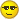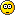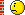-

# Thema: Assemblen und Programmieren klappt, aber.....!

1. ## Assemblen und Programmieren klappt, aber.....!

Anzeige

Bin nun aufmerksam durch das tutorial von microcontroller.net gegangen, und an dem punkt angelangt, wo die erste code geschrieben wird und rübergespielt wird.

mein selbstgebauter ISP funktioniert, sowie auch mein µC (atmel atmega, denn mit ponyprog oder mit yaap wird er als atmega8 erkannt. außerdem im AVR studio wird er natürlich nicht erkannt, aber ich glaube, dass das an meinem ISP liegt.

jedenfalls hab ich den ersten code geschrieben, assembliert und mit ponyprog rübergespielt. der code ist anscheinend auch im µC drin, denn er lässt sich ja immer wieder korrekterweise auslesen!

als ich dann eine led an den PB0 (das ist der ausgang) angeschlossen habe, und über widerstand an vcc gegangen bin, leuchtet diese allerdings nicht, obwohl sie es tun müsste.... [-(

wo könnte hier der fehler sitzen? bin halt etwas neu...dadurch kann ich noch nicht ganz selbst fehleranalyse durchführen!

danke im voraus für eure antwortenZitieren

2. Hast du ein Messgerät zur Verfügung?
Hast du die LED richtig herum angeschlossen?

Gruss, AlexZitieren

3. led ist richtig angeschlossen! hab es auch schon anders herum versucht...

messgerät hab ich gerade versucht, es liegt aber keine spannung an, vielleicht nur so 0,5V...

ich bin verzweifelt.
hier der code, vielleicht liegts daran:
Code:
```.include "4433def.inc"       ;Definitionsdatei einbinden, ggf. durch
;2333def.inc ersetzen

ldi r16, 0xFF       ;0xFF ins Arbeitsregister r16 laden
out DDRB, r16       ;Inhalt von r16 ins IO-Register DDRB ausgeben

ldi r16, 0b11111110 ;0b11111100 in r16 laden
out PORTB, r16      ;r16 ins IO-Register PORTB ausgeben

ende:    rjmp ende           ;Sprung zur Marke "ende" -> Endlosschleife```
und ich habe dann meine led an PB0 angeschlossen, also ganz unten links an den pin des µC (mikrocontroller)Zitieren

4. hi .
gebe mal für die include"4433de.inc die für den mega8 an

mfg tottyZitieren

5. meinste, daran könnte es liegen?

die 4433def.inc
Code:
```;***************************************************************************
;* A P P L I C A T I O N   N O T E   F O R   T H E   A V R   F A M I L Y
;*
;* Number		:AVR000
;* File Name		:"4433def.inc"
;* Title		:Register/Bit Definitions for the AT90S4433
;* Date                 :99.01.28
;* Version              :1.30
;* Support telephone	:+47 72 88 43 88 (ATMEL Norway)
;* Support fax		:+47 72 88 43 99 (ATMEL Norway)
;* Support E-mail	:avr@atmel.com
;* Target MCU		:AT90S4433
;*
;* DESCRIPTION
;* When including this file in the assembly program file, all I/O register
;* names and I/O register bit names appearing in the data book can be used.
;* In addition, the six registers forming the three data pointers X, Y and
;* Z have been assigned names XL - ZH. Highest RAM address for Internal
;* SRAM is also defined
;*
;*
;* The Register Bit names are represented by their bit number (0-7).
;*
;* Please observe the difference in using the bit names with instructions
;* such as "sbr"/"cbr" (set/clear bit in register) and "sbrs"/"sbrc"
;* (skip if bit in register set/cleared). The following example illustrates
;* this:
;*
;* in	r16,PORTB		;read PORTB latch
;* sbr	r16,(1<<PB6)+(1<<PB5)	;set PB6 and PB5 (use masks, not bit#)
;* out  PORTB,r16		;output to PORTB
;*
;* in	r16,TIFR		;read the Timer Interrupt Flag Register
;* sbrc	r16,TOV0		;test the overflow flag (use bit#)
;* rjmp	TOV0_is_set		;jump if set
;* ...				;otherwise do something else
;***** Specify Device
.device AT90S4433

;***** I/O Register Definitions
.equ	SREG	=\$3f
.equ	SP	=\$3d
.equ	SPL	=\$3d
.equ	GIMSK	=\$3b
.equ	GIFR	=\$3a
.equ	TIMSK	=\$39
.equ	TIFR	=\$38
.equ    MCUCR   =\$35
.equ    MCUSR   =\$34
.equ	TCCR0	=\$33
.equ	TCNT0	=\$32
.equ	TCCR1A	=\$2f
.equ	TCCR1B	=\$2e
.equ	TCNT1H	=\$2d
.equ	TCNT1L	=\$2c
.equ	OCR1H	=\$2b
.equ	OCR1L	=\$2a
.equ	ICR1H	=\$27
.equ	ICR1L	=\$26
.equ	WDTCR	=\$21
.equ	EEAR	=\$1e
.equ	EEDR	=\$1d
.equ	EECR	=\$1c
.equ	PORTB	=\$18
.equ	DDRB	=\$17
.equ	PINB	=\$16
.equ	PORTC	=\$15
.equ	DDRC	=\$14
.equ	PINC	=\$13
.equ	PORTD	=\$12
.equ	DDRD	=\$11
.equ	PIND	=\$10
.equ	SPDR	=\$0f
.equ	SPSR	=\$0e
.equ	SPCR	=\$0d
.equ	UDR	=\$0c
.equ	UCSRA 	=\$0b
.equ	UCSRB 	=\$0a
.equ	UBRR	=\$09
.equ	UBRRL	=\$09
.equ	ACSR	=\$08
.equ	UBRRH	=\$03

;***** Bit Definitions

.equ	WDRF	=3
.equ	BORF	=2
.equ	EXTRF	=1
.equ	PORF	=0

.equ	INT1	=7
.equ	INT0	=6

.equ	INTF1	=7
.equ	INTF0	=6

.equ    TOIE1   =7
.equ    OCIE1   =6
.equ    TICIE1  =3
.equ    TOIE0   =1

.equ    TOV1    =7
.equ    OCF1    =6
.equ    ICF1    =3
.equ    TOV0    =1

.equ    SE      =5
.equ    SM      =4
.equ    ISC11   =3
.equ    ISC10   =2
.equ    ISC01   =1
.equ    ISC00   =0

.equ	CS02	=2
.equ	CS01	=1
.equ	CS00	=0

.equ	COM11	=7
.equ	COM10	=6
.equ	PWM11	=1
.equ	PWM10	=0

.equ	ICNC1	=7
.equ	ICES1	=6
.equ	CTC1	=3
.equ	CS12	=2
.equ	CS11	=1
.equ	CS10	=0

.equ	WDTOE	=4
.equ	WDE	=3
.equ	WDP2	=2
.equ	WDP1	=1
.equ	WDP0	=0

.equ    EERIE   =3
.equ	EEMWE	=2
.equ	EEWE	=1
.equ	EERE	=0

.equ	SPIE	=7
.equ	SPE	=6
.equ	DORD	=5
.equ	MSTR	=4
.equ	CPOL	=3
.equ	CPHA	=2
.equ	SPR1	=1
.equ	SPR0	=0

.equ	SPIF	=7
.equ	WCOL	=6

.equ	RXC	=7
.equ	TXC	=6
.equ	UDRE	=5
.equ	FE	=4
.equ	OR	=3
.equ    MPCM    =0

.equ	RXCIE	=7
.equ	TXCIE	=6
.equ	UDRIE	=5
.equ	RXEN	=4
.equ	TXEN	=3
.equ	CHR9	=2
.equ	RXB8	=1
.equ	TXB8	=0

.equ	ACD	=7
.equ	AINBG 	=6
.equ	ACO	=5
.equ	ACI	=4
.equ	ACIE	=3
.equ	ACIC	=2
.equ	ACIS1	=1
.equ	ACIS0	=0

.equ    MUX2    =2
.equ    MUX1    =1
.equ    MUX0    =0

.equ	PB5	=5
.equ	PB4	=4
.equ	PB3	=3
.equ	PB2	=2
.equ	PB1	=1
.equ	PB0	=0

.equ	DDB5	=5
.equ	DDB4	=4
.equ	DDB3	=3
.equ	DDB2	=2
.equ	DDB1	=1
.equ	DDB0	=0

.equ	PINB5	=5
.equ	PINB4	=4
.equ	PINB3	=3
.equ	PINB2	=2
.equ	PINB1	=1
.equ	PINB0	=0

.equ	PC5	=5
.equ	PC4	=4
.equ	PC3	=3
.equ	PC2	=2
.equ	PC1	=1
.equ	PC0	=0

.equ	DDC5	=5
.equ	DDC4	=4
.equ	DDC3	=3
.equ	DDC2	=2
.equ	DDC1	=1
.equ	DDC0	=0

.equ	PINC5	=5
.equ	PINC4	=4
.equ	PINC3	=3
.equ	PINC2	=2
.equ	PINC1	=1
.equ	PINC0	=0

.equ	PD7	=7
.equ	PD6	=6
.equ	PD5	=5
.equ	PD4	=4
.equ	PD3	=3
.equ	PD2	=2
.equ	PD1	=1
.equ	PD0	=0

.equ	DDD7	=7
.equ	DDD6	=6
.equ	DDD5	=5
.equ	DDD4	=4
.equ	DDD3	=3
.equ	DDD2	=2
.equ	DDD1	=1
.equ	DDD0	=0

.equ	PIND7	=7
.equ	PIND6	=6
.equ	PIND5	=5
.equ	PIND4	=4
.equ	PIND3	=3
.equ	PIND2	=2
.equ	PIND1	=1
.equ	PIND0	=0

.def	XL	=r26
.def	XH	=r27
.def	YL	=r28
.def	YH	=r29
.def	ZL	=r30
.def	ZH	=r31

.equ 	RAMEND  =\$DF	;Last On-Chip SRAM Location
.equ	XRAMEND =\$DF
.equ	E2END	=\$FF
.equ	FLASHEND=\$7FFZitieren

6. hat echt einfach niemand einen löäsungsansatz

ich bin so verzweifelt, hab alle grundschaltungen fehlerfrei aufgebaut und nun funktionieren meine i/o-ports des µC nicht (es fließt nur spannung von ca. 0,3V). hab mikrocontroller auch schon ausgewechselt!

ich werf das dings gleich inne ecke!Zitieren

7. Bei dir ist der Name echt Programm ...
Zitat aus der Datei:
;* Target MCU :AT90S4433
Denk mal drüber nach!

Gruss, AlexZitieren

8. boah, soweit bin ich noch nicht den fehlern auf den grund gegangen! brauch jetzt also eine neue *.inc, richtig?Zitieren

9. tja, und die finde ich nicht über google! hätte jemand vielleicht eine *incZitieren

10. sind doch beim AVR-Studio alle dabei, unter AppnotesZitieren

####Berechtigungen

• Neue Themen erstellen: Nein
• Themen beantworten: Nein